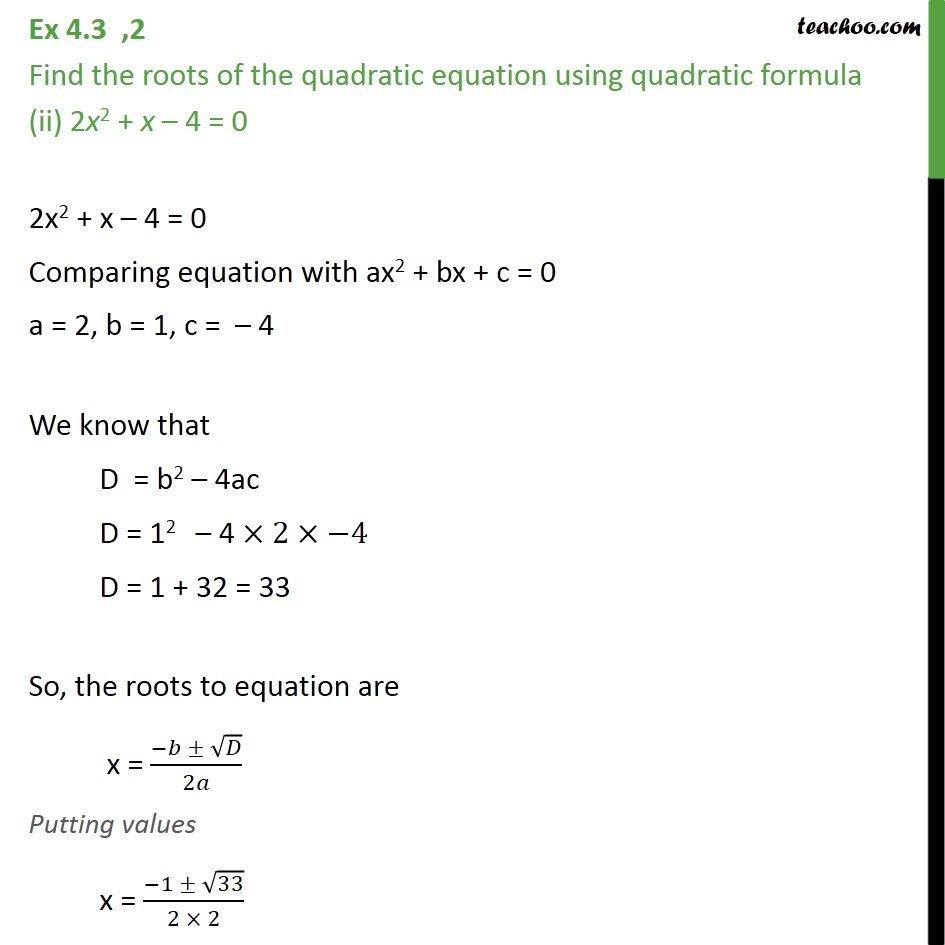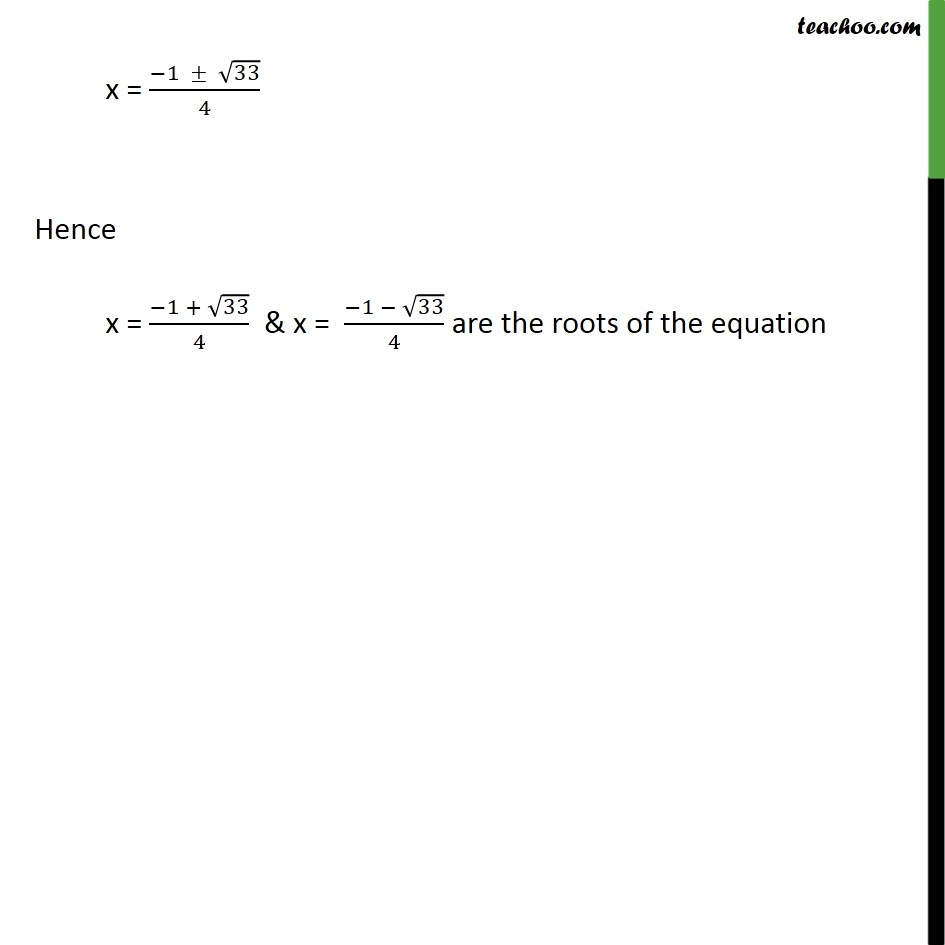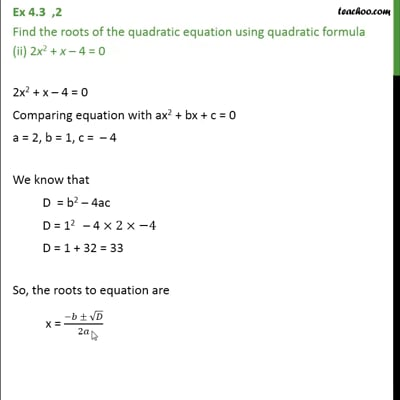Ex 4.3

Chapter 4 Class 10 Quadratic Equations
Serial order wiseThis video is only available for Teachoo black users

Introducing your new favourite teacher - Teachoo Black, at only ₹83 per month

### Transcript

Ex 4.3 ,2 Find the roots of the quadratic equation using quadratic formula (ii) 2x2 + x 4 = 0 2x2 + x 4 = 0 Comparing equation with ax2 + bx + c = 0 a = 2, b = 1, c = 4 We know that D = b2 4ac D = 12 4 2 4 D = 1 + 32 = 33 So, the roots to equation are x = ( )/2 Putting values x = ( 1 33)/(2 2) x = ( 1 33)/4 Hence x = ( 1 + 33)/4 & x = ( 1 33)/4 are the roots of the equation